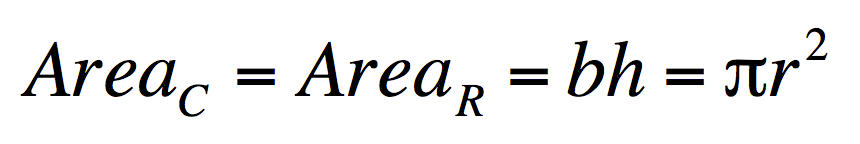# Deriving the formula for area of a circle

A first exposure for a grade 7 student on the basic parts of a circle and the derivation of a circle’s area formula. It was engaging and interactive and went from the concrete to the abstract quite quickly because it was all so visually obvious. There was drawing, outlining, folding, cutting, building, measuring, writing, and a whole lot of problem solving going on. The activity brought understanding and meaning to the formula Ac= πr2. More exposures deriving the area formula will help solidify what was learned but first the brain needs a chance to sort and settle all the new information. Next session we will start applying the area formula to circles of different diameters and circumferences.

Note: a) To reshape the circle in its entirety into a right rectangle we’d need to create an infinite number of triangles within the circle. b) The numbers did not work out exact as the circle we built was not a perfect circle but it was close enough to show the derivation of the formula.

We could come at this from a similar but different approach by inscribing a regular polygon in the circle, cutting the regular polygon up into isosceles triangles, cutting each isosceles triangles into two right triangles, reshaping each pair of right triangles into a rectangle, and finally reshaping the smaller rectangles into a large rectangle.

With an infinite sided regular polygon inscribed in the circle, the circle begins to look like the regular polygon, and the regular polygon begins to look like the circle; the apothem of the polygon becomes the radius of the circle and the perimeter of the polygon becomes the circumference of the circle.

Enjoy playing around with this activity – send photos of your circle activities and I will post them up.

For instructions on how to make the half blocks that were used in this activity please watch the video on the Fractional / Decimal Blocks post.